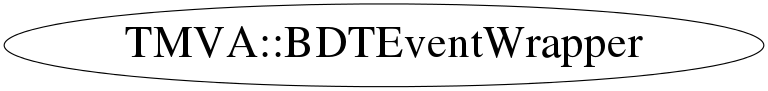# class TMVA::BDTEventWrapper

## Function Members (Methods)

public:
 ~BDTEventWrapper() TMVA::BDTEventWrapper BDTEventWrapper(const TMVA::Event*) TMVA::BDTEventWrapper BDTEventWrapper(const TMVA::BDTEventWrapper&) Double_t GetCumulativeWeight(Bool_t type) const Double_t GetVal() const const TMVA::Event* operator*() const Bool_t operator<(const TMVA::BDTEventWrapper& other) const TMVA::BDTEventWrapper& operator=(const TMVA::BDTEventWrapper&) void SetCumulativeWeight(Bool_t type, Double_t weight) static void SetVarIndex(Int_t iVar)
private:
 static Int_t& GetVarIndex()

## Data Members

private:
 Double_t fBkgWeight cumulative background weight for splitting const TMVA::Event* fEvent pointer to the event Double_t fSigWeight same for the signal weights

## Class Charts## Function documentation

BDTEventWrapper(const Event* e)
``` constuctor
```

``` destructor
```
void SetCumulativeWeight(Bool_t type, Double_t weight)
``` Set the accumulated weight, for sorted signal/background events

* @param fType - true for signal, false for background
* @param weight - the total weight

```
Double_t GetCumulativeWeight(Bool_t type) const
``` Get the accumulated weight
```
Bool_t operator<(const TMVA::BDTEventWrapper& other) const
BDTEventWrapper( const Event* )
void SetVarIndex(Int_t iVar)
``` Set the index of the variable to compare on

* @param iVar - index of the variable in fEvent to use

```
`{ if (iVar >= 0) GetVarIndex() = iVar; }`
Double_t GetVal() const
``` Return the value of variable fVarIndex for this event

* @return value of variable fVarIndex for this event

```
`{ return fEvent->GetValue(GetVarIndex()); }`
const Event* operator*() const
`{ return fEvent; }`

``` This is a workaround for OSx where static thread_local data members are
not supported. The C++ solution would indeed be the following:
```
`{TTHREAD_TLS(Int_t) fVarIndex(0); return fVarIndex;}`